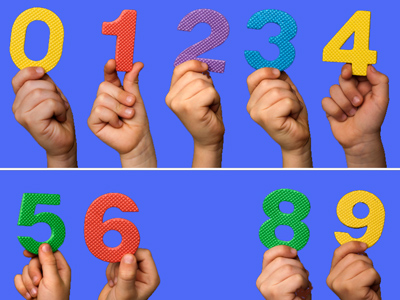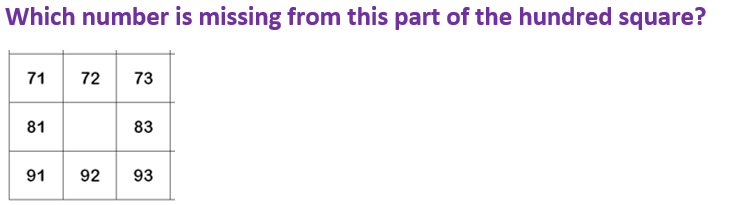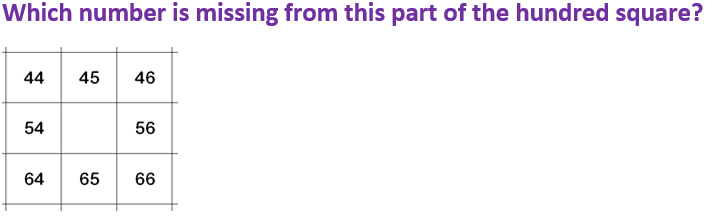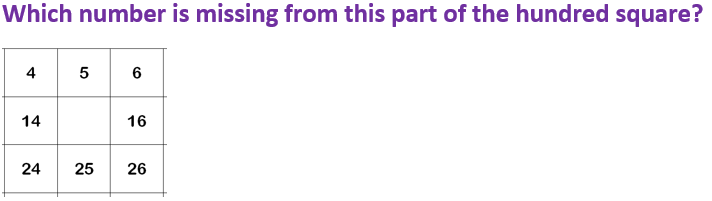Being able to recognise the missing number in a sequence is vitally important in maths.

# Year 2 Numbers - Place Value Problem Solving

This quiz addresses the requirements of the National Curriculum KS1 Maths and Numeracy for children aged 6 and 7 in year 2. Specifically this quiz is aimed at the section dealing with using place value and number facts to solve problems.

Children in Year 2 begin to learn about what each digit in a number represents, or its place value. For example, in the number 34, there are 3 tens and 4 ones. They can use this knowledge of place values to solve problems using 100 squares, answer more than/less than questions, solve money problems and also use it to answer number bond questions.

Question 1
Which number is 4 more than 65?
66
69
79
80
‘4 more’ means adding 4 to 65
Question 2
Which number is missing from this number sentence?
20 - ? = 13
17
23
7
13
13 + 7 = 20, so 20 – 7 must be 13!
Question 385
82
38
88
82 is one less than 83, and ten less than 92
Question 455
85
59
32
55 is one more than 54, and 10 more than 45
Question 56
15
23
27
15 is one less than 16 and ten more than 5
Question 6
Which number is missing from this number sentence?
12 + ? = 20
8
18
12
10
Knowing numbers bonds or pairs to 20 helps here
Question 7
Which number is 20 less than 80?
60
67
63
70
’20 less’ means counting back 20 from 80, or subtracting 20
Question 8
Which number is 10 more than 43?
44
54
63
53
‘10 more’ means adding 10 to the number
Question 9
Kate has 42p. Joe has 10p more. How much does Joe have?
62p
52p
32p
22p
10p more means adding one 10 in the tens column
Question 10
I have £1 and spend 50p. How much do I have left?
80p
20p
60p
50p
£1 is 100p. 50p less than this is 50p
Author:  Angela Smith